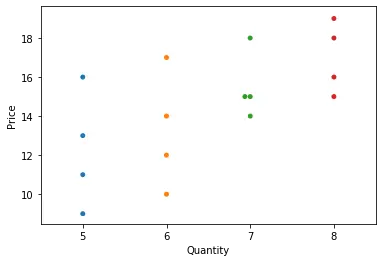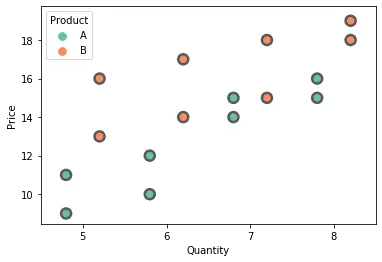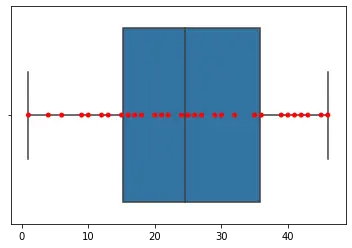# Seaborn Swarmplot

Manav Narula Oct 12, 2021

A scatter plot is one of the most basic and useful graphs used. The seaborn module in Python can take such graphs a step further and use them to represent categorical values.

A swarm plot is a type of scatter plot that is used for representing categorical values. It is very similar to the strip plot, but it avoids the overlapping of points. We can use the `seaborn.swarmplot()` to create such graphs.

It is not advisable to use this type of graph when the sample size is large.

We will use the `swarmplot()` function to create such a graph for categorical values in the following code.

``````import pandas as pd
import matplotlib.pyplot as plt
import seaborn as sns

df = pd.DataFrame(
{
"Quantity": [5, 6, 7, 8, 5, 6, 7, 8, 5, 6, 7, 8, 5, 6, 7, 8],
"Price": [9, 10, 15, 16, 13, 14, 15, 18, 11, 12, 14, 15, 16, 17, 18, 19],
"Day": [1, 1, 1, 1, 1, 1, 1, 1, 2, 2, 2, 2, 2, 2, 2, 2],
"Product": [
"A",
"A",
"A",
"A",
"B",
"B",
"B",
"B",
"A",
"A",
"A",
"A",
"B",
"B",
"B",
"B",
],
}
)

sns.swarmplot(data=df, y="Price", x="Quantity")
``````In the above example, we can add the `hue` parameter to the `swarmplot()` function and separate the categories for the two different products. We can split the two products using the `dodge` argument and setting it to True.

Additionally, we can use some other arguments also with the `swarmplot()` function. The size and color of the points can be changed using the `size` and `color` arguments. We can alter the color combination used for different categories using the `palette` parameter. The `linewidth` argument adds a border to the points of the specified width. The order of the categories plotted can be controlled using the `order` parameter.

We will try to make use of the above arguments in the following example.

``````import pandas as pd
import matplotlib.pyplot as plt
import seaborn as sns

df = pd.DataFrame(
{
"Quantity": [5, 6, 7, 8, 5, 6, 7, 8, 5, 6, 7, 8, 5, 6, 7, 8],
"Price": [9, 10, 15, 16, 13, 14, 15, 18, 11, 12, 14, 15, 16, 17, 18, 19],
"Day": [1, 1, 1, 1, 1, 1, 1, 1, 2, 2, 2, 2, 2, 2, 2, 2],
"Product": [
"A",
"A",
"A",
"A",
"B",
"B",
"B",
"B",
"A",
"A",
"A",
"A",
"B",
"B",
"B",
"B",
],
}
)

sns.swarmplot(
data=df,
y="Price",
x="Quantity",
hue="Product",
dodge=True,
linewidth=2.5,
palette="Set2",
size=10,
)
``````The `swarmplot()` can also be used as a good compliment over a box or violin plot. It gives a good idea of the distribution of the data.

We can add a box plot over the swarm plot using the following code.

``````import random
import numpy as np
import seaborn as sns

n = random.sample(range(0, 50), 30)
arr = np.array(n)
sns.boxplot(n)
sns.swarmplot(n, color="red")
``````The `catplot()` function can also create such a swarm plot. For this, we need to specify the value for the `kind` parameter in the `catplot()` function as `swarm`.

Author: Manav Narula

Manav is a IT Professional who has a lot of experience as a core developer in many live projects. He is an avid learner who enjoys learning new things and sharing his findings whenever possible.